Related Articles

# Karger’s algorithm for Minimum Cut | Set 1 (Introduction and Implementation)

• Difficulty Level : Hard
• Last Updated : 24 Jun, 2021

Given an undirected and unweighted graph, find the smallest cut (smallest number of edges that disconnects the graph into two components).
The input graph may have parallel edges.
For example consider the following example, the smallest cut has 2 edges.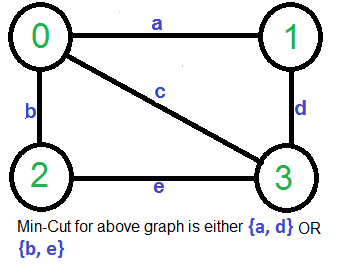A Simple Solution use Max-Flow based s-t cut algorithm to find minimum cut. Consider every pair of vertices as source ‘s’ and sink ‘t’, and call minimum s-t cut algorithm to find the s-t cut. Return minimum of all s-t cuts. Best possible time complexity of this algorithm is O(V5) for a graph. [How? there are total possible V2 pairs and s-t cut algorithm for one pair takes O(V*E) time and E = O(V2)].
Below is simple Karger’s Algorithm for this purpose. Below Karger’s algorithm can be implemented in O(E) = O(V2) time.

```1)  Initialize contracted graph CG as copy of original graph
2)  While there are more than 2 vertices.
a) Pick a random edge (u, v) in the contracted graph.
b) Merge (or contract) u and v into a single vertex (update
the contracted graph).
c) Remove self-loops
3) Return cut represented by two vertices.```

Let us understand above algorithm through the example given.
Let the first randomly picked vertex be ‘a‘ which connects vertices 0 and 1. We remove this edge and contract the graph (combine vertices 0 and 1). We get the following graph.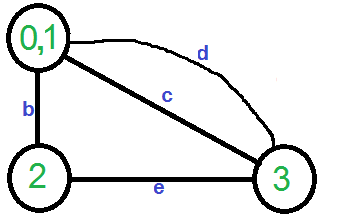Let the next randomly picked edge be ‘d’. We remove this edge and combine vertices (0,1) and 3.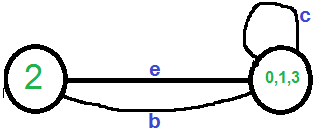We need to remove self-loops in the graph. So we remove edge ‘c’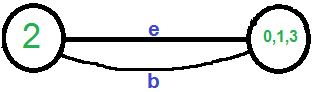Now graph has two vertices, so we stop. The number of edges in the resultant graph is the cut produced by Karger’s algorithm.
Karger’s algorithm is a Monte Carlo algorithm and cut produced by it may not be minimum. For example, the following diagram shows that a different order of picking random edges produces a min-cut of size 3.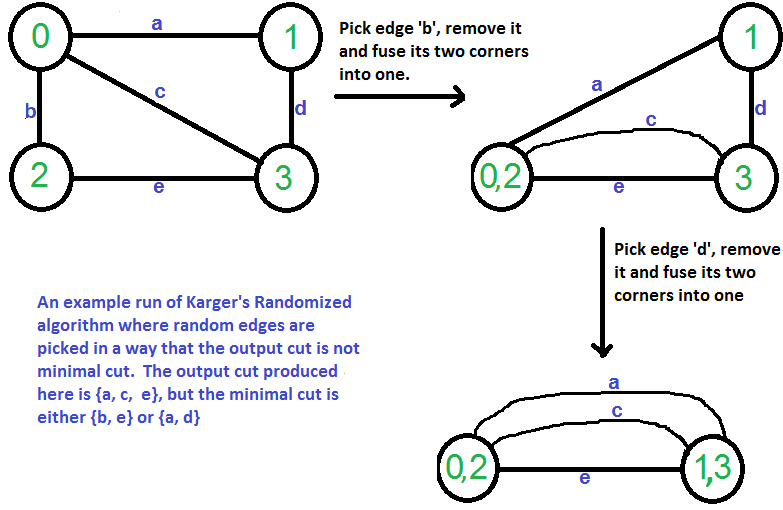Below is C++ implementation of above algorithm. The input graph is represented as a collection of edges and union-find data structure is used to keep track of components.

## C

 `// Karger's algorithm to find Minimum Cut in an``// undirected, unweighted and connected graph.``#include ``#include ``#include ` `// a structure to represent a unweighted edge in graph``struct` `Edge``{``    ``int` `src, dest;``};` `// a structure to represent a connected, undirected``// and unweighted graph as a collection of edges.``struct` `Graph``{``    ``// V-> Number of vertices, E-> Number of edges``    ``int` `V, E;` `    ``// graph is represented as an array of edges.``    ``// Since the graph is undirected, the edge``    ``// from src to dest is also edge from dest``    ``// to src. Both are counted as 1 edge here.``    ``Edge* edge;``};` `// A structure to represent a subset for union-find``struct` `subset``{``    ``int` `parent;``    ``int` `rank;``};` `// Function prototypes for union-find (These functions are defined``// after kargerMinCut() )``int` `find(``struct` `subset subsets[], ``int` `i);``void` `Union(``struct` `subset subsets[], ``int` `x, ``int` `y);` `// A very basic implementation of Karger's randomized``// algorithm for finding the minimum cut. Please note``// that Karger's algorithm is a Monte Carlo Randomized algo``// and the cut returned by the algorithm may not be``// minimum always``int` `kargerMinCut(``struct` `Graph* graph)``{``    ``// Get data of given graph``    ``int` `V = graph->V, E = graph->E;``    ``Edge *edge = graph->edge;` `    ``// Allocate memory for creating V subsets.``    ``struct` `subset *subsets = ``new` `subset[V];` `    ``// Create V subsets with single elements``    ``for` `(``int` `v = 0; v < V; ++v)``    ``{``        ``subsets[v].parent = v;``        ``subsets[v].rank = 0;``    ``}` `    ``// Initially there are V vertices in``    ``// contracted graph``    ``int` `vertices = V;` `    ``// Keep contracting vertices until there are``    ``// 2 vertices.``    ``while` `(vertices > 2)``    ``{``       ``// Pick a random edge``       ``int` `i = ``rand``() % E;` `       ``// Find vertices (or sets) of two corners``       ``// of current edge``       ``int` `subset1 = find(subsets, edge[i].src);``       ``int` `subset2 = find(subsets, edge[i].dest);` `       ``// If two corners belong to same subset,``       ``// then no point considering this edge``       ``if` `(subset1 == subset2)``         ``continue``;` `       ``// Else contract the edge (or combine the``       ``// corners of edge into one vertex)``       ``else``       ``{``          ``printf``(``"Contracting edge %d-%d\n"``,``                 ``edge[i].src, edge[i].dest);``          ``vertices--;``          ``Union(subsets, subset1, subset2);``       ``}``    ``}` `    ``// Now we have two vertices (or subsets) left in``    ``// the contracted graph, so count the edges between``    ``// two components and return the count.``    ``int` `cutedges = 0;``    ``for` `(``int` `i=0; i subsets[yroot].rank)``        ``subsets[yroot].parent = xroot;` `    ``// If ranks are same, then make one as root and``    ``// increment its rank by one``    ``else``    ``{``        ``subsets[yroot].parent = xroot;``        ``subsets[xroot].rank++;``    ``}``}` `// Creates a graph with V vertices and E edges``struct` `Graph* createGraph(``int` `V, ``int` `E)``{``    ``Graph* graph = ``new` `Graph;``    ``graph->V = V;``    ``graph->E = E;``    ``graph->edge = ``new` `Edge[E];``    ``return` `graph;``}` `// Driver program to test above functions``int` `main()``{``    ``/* Let us create following unweighted graph``        ``0------1``        ``| \    |``        ``|   \  |``        ``|     \|``        ``2------3   */``    ``int` `V = 4;  ``// Number of vertices in graph``    ``int` `E = 5;  ``// Number of edges in graph``    ``struct` `Graph* graph = createGraph(V, E);` `    ``// add edge 0-1``    ``graph->edge.src = 0;``    ``graph->edge.dest = 1;` `    ``// add edge 0-2``    ``graph->edge.src = 0;``    ``graph->edge.dest = 2;` `    ``// add edge 0-3``    ``graph->edge.src = 0;``    ``graph->edge.dest = 3;` `    ``// add edge 1-3``    ``graph->edge.src = 1;``    ``graph->edge.dest = 3;` `    ``// add edge 2-3``    ``graph->edge.src = 2;``    ``graph->edge.dest = 3;` `    ``// Use a different seed value for every run.``    ``srand``(``time``(NULL));` `    ``printf``(``"\nCut found by Karger's randomized algo is %d\n"``,``           ``kargerMinCut(graph));` `    ``return` `0;``}`

Output:

```Contracting edge 0-2
Contracting edge 0-3

Cut found by Karger's randomized algo is 2```

Note that the above program is based on outcome of a random function and may produce different output.
In this post, we have discussed simple Karger’s algorithm and have seen that the algorithm doesn’t always produce min-cut. The above algorithm produces min-cut with probability greater or equal to that 1/(n2). See next post on Analysis and Applications of Karger’s Algorithm, applications, proof of this probability and improvements are discussed.
References:
http://en.wikipedia.org/wiki/Karger%27s_algorithm